# Czech crowns

Small Petra save money. Grandfather give her 10 crowns. Petra asked his older sister let her calculate how much he has saved up. Sister told her that he has less than 30 crowns. If the sum is divided by three left one crown. If the sum is divided by the five left with her two. How much Petra has saved up?

x =  22

### Step-by-step explanation:

10 Kč: 10 = 3 × 3 + 1;     10 = 2 × 5 + 0

11 Kč: 11 = 3 × 3 + 2;     11 = 2 × 5 + 1

12 Kč: 12 = 4 × 3 + 0;     12 = 2 × 5 + 2

13 Kč: 13 = 4 × 3 + 1;     13 = 2 × 5 + 3

14 Kč: 14 = 4 × 3 + 2;     14 = 2 × 5 + 4

15 Kč: 15 = 5 × 3 + 0;     15 = 3 × 5 + 0

16 Kč: 16 = 5 × 3 + 1;     16 = 3 × 5 + 1

17 Kč: 17 = 5 × 3 + 2;     17 = 3 × 5 + 2

18 Kč: 18 = 6 × 3 + 0;     18 = 3 × 5 + 3

19 Kč: 19 = 6 × 3 + 1;     19 = 3 × 5 + 4

20 Kč: 20 = 6 × 3 + 2;     20 = 4 × 5 + 0

21 Kč: 21 = 7 × 3 + 0;     21 = 4 × 5 + 1

22 Kč: 22 = 7 × 3 + 1;     22 = 4 × 5 + 2 ⇐⇐⇐⇐

23 Kč: 23 = 7 × 3 + 2;     23 = 4 × 5 + 3

24 Kč: 24 = 8 × 3 + 0;     24 = 4 × 5 + 4

25 Kč: 25 = 8 × 3 + 1;     25 = 5 × 5 + 0

26 Kč: 26 = 8 × 3 + 2;     26 = 5 × 5 + 1

27 Kč: 27 = 9 × 3 + 0;     27 = 5 × 5 + 2

28 Kč: 28 = 9 × 3 + 1;     28 = 5 × 5 + 3

29 Kč: 29 = 9 × 3 + 2;     29 = 5 × 5 + 4

30 Kč: 30 = 10 × 3 + 0;     30 = 6 × 5 + 0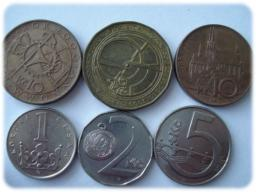We will be pleased if You send us any improvements to this math problem. Thank you!## Related math problems and questions:

• Czech crownsOldrich has one crown. Peter has five crowns coin, a two crown coin and a one-crown coin. Radek has twenty-crown banknote, ten banknote and five-crown coin. The boys got one fifty-one crown and one crown coin. How can they share the money fairly when they
• Crown coinsJana saves two-crown and five-crown coins. She has ten coins in the cashier. How many two crowns and how many five crowns shw have if she saved 29 crowns?
• Crown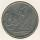Siblings collect a 2-crowns and 5-crowns. Together have 80 coins with a total of value 310 crowns. How many saved two-crowns and how many five-crowns?
• GroupA group of kids wanted to ride. When the children were divided into groups of 3 children, one remain. When divided into groups of 4 children, 1 remains. When divided into groups of 6 children, 1 remains. After divided into groups of 5 children, no one lef
• Brothers and sisters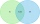There are 35 children in the class, 23 of them have a brother, and 27 of them have a sister. How many children have both a brother and a sister when there are 5 children in the class who have no brother or sister?
• SavingAt the beginning of the year, Tomas had saved 230kc, after half a year even 590 CZK. How many crowns did he save each month when saving the same amount?
• April (month)April has 30 days. Roman saves his money in the box for the whole month. Every third day you saves 10 cents. How many cents did Roman saved during the whole month when start saving on April 1?
• Gloves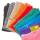Petra has ten pairs of gloves in the closet. Six pairs are blue, 4 pairs are yellow. How many pieces of gloves need to be pulled out, at least when she pulls them out in the dark and wants to have one complete one-color pair?
• Dort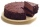The cube-shaped cube box has the dimensions of 30 cm × 30 cm × 12 cm. How many CZK (Czech crowns) would cost be the biggest cake that would fit into the box when a 10 cm³ cake costs CZK 0.5? The cake has the same shape as the box.
• Brother and sisterWhen I was 8, my sister was 4, and now I am 18. How old is my sister?
• Johnny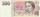Johnny bought for 28 CZK flower to mum. He that spent 4/7 of the money saved. How much money he had saved? How much money left him?
• Coins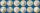Harvey had saved up a number of 2-euro coins. He stored coins in a single layer in a square. Left 6 coins. When he make square, which has one more row, missing 35 coins. How many euros he have?
• Number train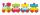The numbers 1,2,3,4,5,6,7,8 and 9 traveled by train. The train had three cars and each was carrying just three numbers. No. 1 rode in the first carriage, and in the last carriage was all odd numbers. The conductor calculated sum of the numbers in the firsAdam has half the money in his right pocket than in his left pocket. If he transferred 40 crowns from the left pocket to the right, he would have the same in both pockets. Calculate how many crowns does Adam has in his left pocket more than in his right?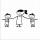Three siblings had saved up a total of 1,274 CZK. Peter had saved up to 15% more than Jirka and Hanka 10% less than Peter. How much money did they save each one of them?Vilem, Cenek, and Edita divided the money they earned by spreading the leaflet. Vilem got 240 CZK more than Cenek and twice more than Edita. Edita got 400kc less than Vilem.Find the smallest integer: divided by 2, the remainder is 1. divided by 3, the remainder is 2. divided by 4, the remainder is 3. ... divided by eight, the remainder is 7, divided by 9 the remainder is 8.